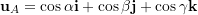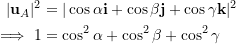### Vectors and their Operations: Cartesian vector notation

### Cartesian coordinate systems

A convenient set of directions is a set of perpendicular directions called orthogonal axes. Orthogonal means perpendicular.  The positive direction of an axis sets a benchmark to determine the positive (or negative) direction of a vector along (parallel with) the axis. For example, considering an axis x shown in Fig. 2.14a,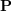is in the positive direction and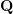is in the negative direction with respect to axis x.

Decomposing a vector in the two-dimensional plane needs two orthogonal axes (Fig. 2.14b). Decomposing a spatial vector (a vector in the three-dimensional space) needs three orthogonal axes (Fig. 2.14c). A set of orthogonal axes, intersecting at a point (the origin), is called a Cartesian coordinate system or Cartesian frame.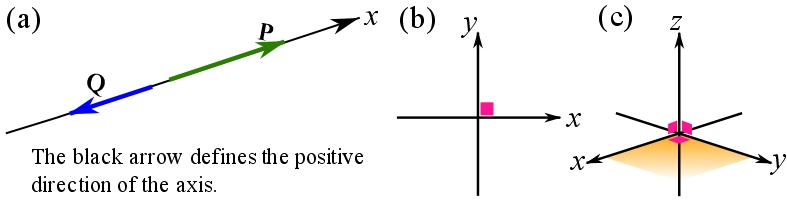Fig. 2.14 (a) Definition of an axis with its positive direction. Two vectors in the positive and negative directions of the axis are demonstrated, (b) a two-dimensional Cartesian coordinate system, (b) a three-dimensional Cartesian coordinate system.

### Unit vector

A Unit vector. A unit vector is a vector of magnitude. Any vector can be written as a scalar multiplication of a unit vector with the same direction as the original vector: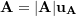such that the notation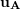indicates the unit vector (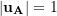) in the direction of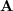. Any vector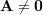can become a unit vector if scaled by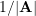meaning that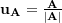. The vectoris called the unit vector of. Making a unit vector out of a vector is called normalizing a vector. The unit vector of a vector has the same direction as the original vector.

Experiment with the following interactive tool to investigate how a vector is a scalar multiplicand of its unit vector.

### Cartesian vector notation

The components of a vector along orthogonal axes are called rectangular components or Cartesian components. A vector decomposed (resolved) into its rectangular components can be expressed by using two possible notations namely the scalar notation (scalar components) and the Cartesian vector notation. Both notations are explained for the two-dimensional (planar) conditions, and then extended to three dimensions in the following sections.

#### Rectangular vector components of coplanar vectors

Consider a vector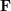and its rectangular components resolved in a Cartesian system as shown in Fig. 2.15. Theand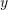vector components ofare resolved and denoted byand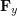respectively. The subscriptsanddenote the axes with which the components are parallel. The magnitude of the vector components are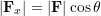and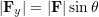. By definition, the magnitudes of a vector is a positive number. Therefore, the magnitude of a vector component does not hold any information about the sense of direction. To include the information about the directions of the vector components in a Cartesian system, scalar notation or scalar components are defined. Scalar components of a vector are signed magnitudes of its rectangular components. A scalar component is positive if the vector component is directed along the positive axis, and negative if the vector component is directed along the negative axis (opposite of the axis positive direction). For the vector componentsandshown in Fig. 2.15, the scalar components are denoted by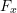and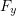. Note the regular and italic font face used for the notation. The scalar components of the vector shown in Fig. 2.15a are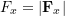and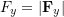both being positive. The scalar components of the vector shown in Fig. 2.15b, however, areand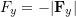. The scalar componentis negative, because its vector componentis directed along the negative direction of the y axis.

An axis has an associated unit vector showing the positive direction of the axis. Any vector parallel with an axis can be written as a scalar multiplier of the axis’ unit vector. The scalar multiplier is equal to the scalar component (signed magnitude) of the vector parallel with the axis. The scalar multiplier is positive if the vector is in the direction of the axis unit vector, and negative otherwise.

The unit vectors associated with Cartesian x and y axes are denoted by bold lower-case lettersandrespectively and their respective small arrows are displayed on the Cartesian axes (Fig. 2.16). Another possible notations areand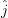.

The rectangular components of any vectorcan be now expressed in terms of the Cartesian axis unit vectors,

(2.1a)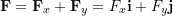whereandare the scalar components.

As a general rule any vectorcan be written as,

(2.1b)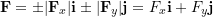Remark: using CVN is equivalent to resolving a vector in a Cartesian coordinate system.

Remark: in CVN, capital letters (non-bold), such as,and, represent scalar components. Their values can be negative or positive, depending on the directions of the components relative to,, and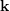. In the formulations of later chapter, where CVN is not used, capital letters represent the magnitudes of vector or vector components, for simplicity.

Remark: components of a vector are vectors, whereas the scalar components (vector coordinates) are scalar (i.e. signed magnitudes).

Using the following interactive tool, you can observe the (vector) components and scalar components of a vector. Observe how the vector is represented in CVN. Change the direction of the vector by the angle slider and observe the values of the scalar components. When do the scalar components attain negative values?

#### EXAMPLE 2.4.1

Determine the scalar components ofshown in the figure below and write the the vectorin CVN.

#### SOLUTIONRemark: the location of a vector with respect to the origin of the Cartesian coordinate system does not affect its Cartesian components. You can always consider (draw) parallel axes with the Cartesian axes at the tail of a vector and calculate its components.

It is worthy to note the relationships among the scalar components, the magnitude, and the direction of a vector. Consider a Cartesian coordinate system in which angles are measured counterclockwise from the positive x axis as demonstrated in Fig. 2.17. Then the following equations hold,

(2.2)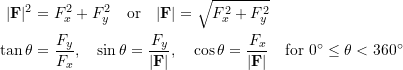To facilitate calculations, specifying the direction of a planar vector in the Cartesian frame can also use the (acute) angle that the vector makes with any of the Cartesian axes. If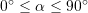and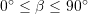are angles of a vector with x and y axes respectively, the following equations hold,

(2.3)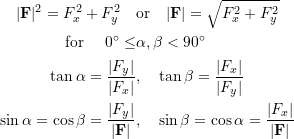Note that the absolute values of the scalar components, which are the magnitudes of the vector Cartesian components, are used in the trigonometry functions. Fig. 2.18a demonstrates three cases of vector decomposition based on the defined anglesand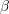. Pay attention to the signs of the scalar components based on the Cartesian components of the vectors.

Another possible way of specifying the direction of a planar vector is by a small slope triangle. The small slope triangle conveys the information on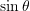,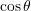, and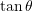of the angle that the vector makes with an axis (Fig. 2.18b).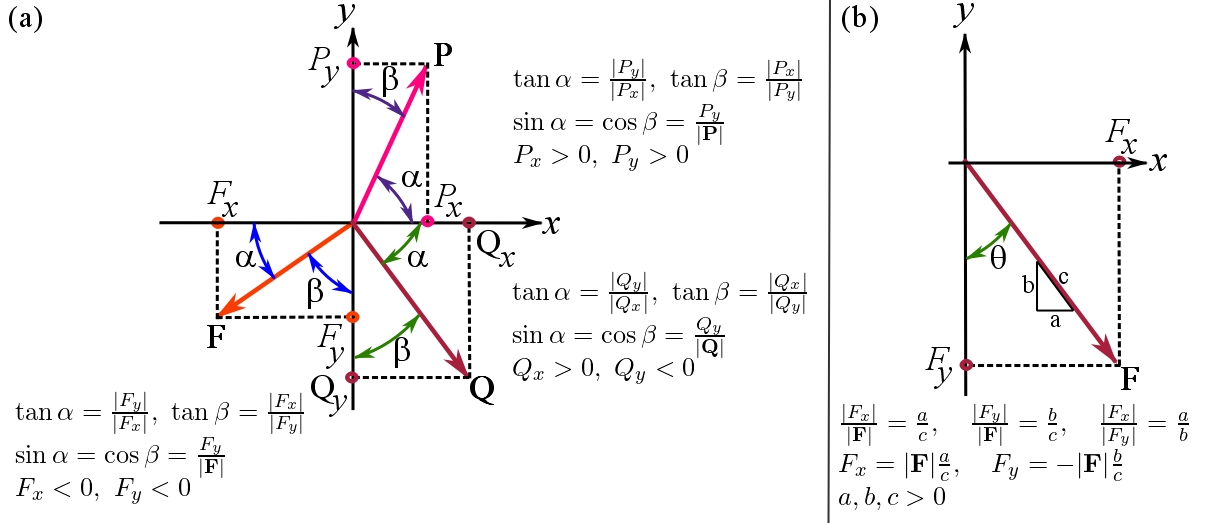Fig. 2.18 The relationships among the scalar components, magnitude, and direction of a vector when the angles of a planar vector with the Cartesian axes are used.

Remark: be cautious about the way the direction of a vector is specified and the proper formulation you should use for the calculations.

Remark: using the angles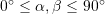(which a vector makes with the Cartesian axes) to specify the direction of the vector has a calculation advantage that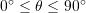is in the domain of the inverse trigonometric functions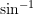,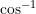and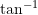.

#### Rectangular vector components of spatial vectors

To treat a vector in three dimensions, a three-dimensional Cartesian coordinate system is to be defined. A common three-dimensional Cartesian coordinate system is a right-handed coordinate system. A coordinate system of three orthogonal axes is said to be right-handed if your right-hand thumb points in the positivedirection when fingers curl from the (positive) x axis to the (positive) y axis (Fig. 2.19a). Another way to define a right-handed Cartesian coordinate system is to follow the right-hand three-finger rule as demonstrated Fig. 2.19b. In three dimensions, the unit vectors of the axes are denoted by,, andas demonstrated in Fig. 2.19c.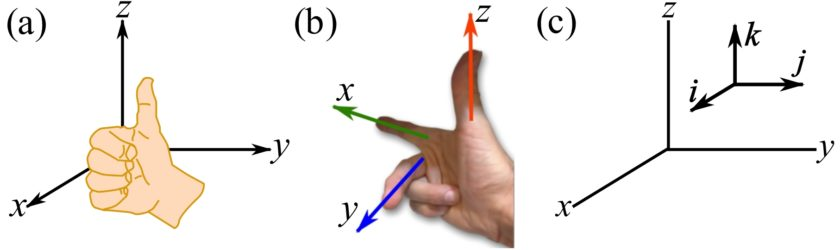Fig. 2.19 (a), (b) A right-handed Cartesian coordinate system, (c) unit vectors of a right-handed Cartesian coordinate system.

Right-click and rotate the following three-dimensional right-handed Cartesian coordinate system to observe its different orientations.

Any vector in three dimensions can be resolved into three rectangular components. A vector expressed in CVN (three dimensions) is written as,

(2.4)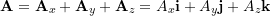where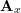,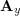and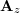are the vector (rectangular or Cartesian) components, and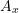,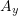and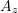are the scalar components (Fig. 2.20a). The magnitude ofis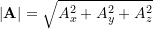. This can be proved by considering the shaded triangles in Fig. 2.20b and twice using the Pythagoras’ theorem.

The direction ofin a three-dimensional Cartesian coordinate system can be defined by the coordinate direction angles,, and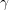measured from the positive x, y, and z axes respectively to the vector (Fig. 2.20c). These angles are limited as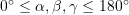. The following relationship holds between the vector magnitude, the scalar components and the coordinate direction angles,

(2.5)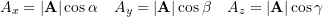The following interactive example illustrates the rectangular components of a vector in three dimensions. Change the scalar components (coordinates of the head point) by the sliders to observe how the vector is expressed in CVN.

The coordinate direction angles of a vector are demonstrated by the following interactive example.

The three coordinate direction angles are not independent and they are related by this equation,

(2.6)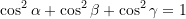which means that knowing two of the angles, the third one is readily obtainable.

To prove Eq. 2.6, a vectoris expressed by its Cartesian components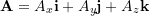and divided by its magnitude,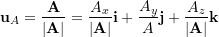which, by Eq. 2.5, is written as: